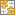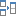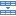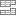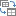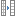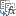# Excel TRANSPOSE function to rotate data from columns to rows

The tutorial explains the syntax of the TRANSPOSE function and shows how to use it correctly to transpose data in Excel.

There's no accounting for tastes. It is also true for work habits. Some Excel users prefer organizing data vertically in columns while others choose horizontal arrangement in rows. In situations when you need to quickly change the orientation of a given range, TRANSPOSE is the function to use.

## Excel TRANSPOSE function - syntax

The purpose of the TRANSPOSE function in Excel is to convert rows to columns, i.e. switch the orientation of a given range from horizontal to vertical or vice versa.

The function takes just one argument:

=TRANSPOSE(array)

Where array is the range of cells to transpose. The array is rotated in this way: the first row of the original array becomes the first column of the new array, the second row becomes the second column, and so on.

Important note! For the TRANSPOSE function to work, you must enter it as an array formula by pressing Ctrl + Shift + Enter.

## How to use the TRANSPOSE function in Excel

The syntax of TRANSPOSE leaves no room for mistakes when building a formula. A trickier part is to enter it correctly in a worksheet. If you don't have much experience with Excel formulas in general and array formulas in particular, please make sure you follow the below steps closely.

### 1. Count the number of columns and rows in the original table

For starters, find out how many columns and rows your source table contains. You will need these numbers in the next step.

In this example, we are going to transpose the table that shows the volume of fresh fruit exports by county: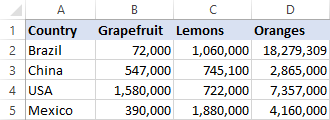Our source table has 4 columns and 5 rows. Keeping these figures in mind, proceed to the next step.

### 2. Select the same number of cells, but change the orientation

Your new table will contain the same number of cells but will be rotated from the horizontal orientation to vertical or vice versa. So, you select a range of empty cells that occupies the same number of rows as the original table has columns, and the same number of columns as the original table has rows.

In our case, we select a range of 5 columns and 4 rows: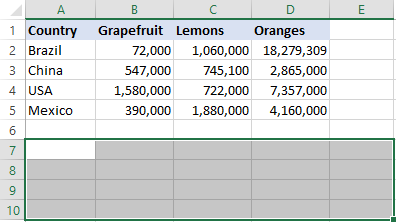### 3. Type the TRANSPOSE formula

With a range of blank cells selected, type the Transpose formula:

`=TRANSPOSE(A1:D5)`

Here are the detailed steps:

First, you type the equality sign, function name and opening parenthesis: =TRANSPOSE(

Then, select the source range using the mouse or type it manually: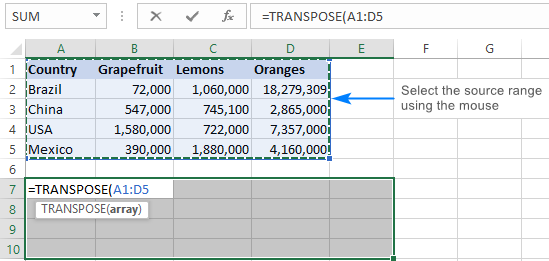Finally, type the closing parenthesis, but don't hit the Enter key! At this point, your Excel Transpose formula should look similar to this: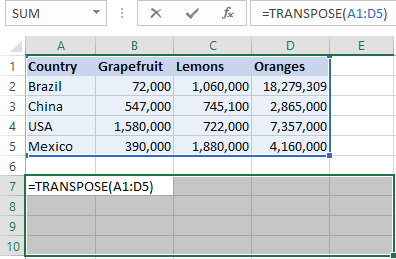### 4. Complete the TRANSPOSE formula

Press Ctrl + Shift + Enter to finish your array formula properly. Why do you need this? Because the formula is to be applied to more than one cell, and it is exactly what array formulas are purposed for.

Once you press Ctrl + Shift + Enter, Excel will surround your Transpose formula with {curly braces} that are visible in the formula bar and are a visual indication of an array formula. In no case you should type them manually, that won't work.

The screenshot below shows that our source table was transposed successfully and 4 columns were converted into 4 rows: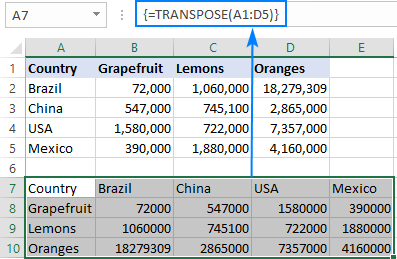## How to transpose data in Excel without zeros for blanks

If one or more cells in the original table is empty, those cells will have zero values in the transposed table, as shown in the screenshot below: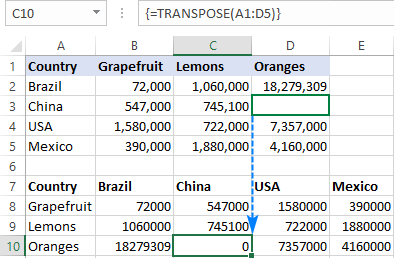If you wish to return blank cells instead, nest the IF function inside your TRANSPOSE formula to check whether a cell is blank or not. If the cell is blank, IF will return an empty string (""), otherwise supply the value to transpose:

`=TRANSPOSE(IF(A1:D5="","",A1:D5))`

Enter the formula as explained above (please remember to press Ctrl + Shift + Enter to finish the array formula correctly), and you will have a result similar to this:## Tips and notes on using TRANSPOSE in Excel

As you have just seen, the TRANSPOSE function has a number of quirks that may confuse unexperienced users. The below tips will help you avoid typical mistakes.

### 1. How to edit a TRANSPOSE formula

As an array function, TRANSPOSE does not allow changing part of the array it returns. To edit a Transpose formula, select the entire range the formula refers to, make the desired change, and press Ctrl + Shift + Enter to save the updated formula.

### 2. How to delete a TRANSPOSE formula

To remove a Transpose formula from your worksheet, select the whole range referenced in the formula, and press the Delete key.

### 3. Replace TRANSPOSE formula with values

When you flip a range by using the TRANSPOSE function, the source range and the output range get linked. This means that whenever you change some value in the original table, the corresponding value in the transposed table changes automatically.

If you'd like to break the connection between the two tables, replace the formula with calculated values. For this, select all the values returned by your formula, press Ctrl + C to copy them, right-click, and choose Paste Special > Values from the context menu.

## Other ways to transpose data in Excel

The TRANSPOSE function is the most popular but not the only way to change columns to rows in Excel. Depending on your particular task, you may want to use one of the following methods:

• If you avoid using array formulas in your worksheets, combine the INDIRECT and ADDRESS functions to rotate a range with a normal non-array formula as shown in this example.
• For automatic column-to-row conversions, the following macro can come in handy: How to transpose in Excel with VBA.
• To perform a one-time conversion without creating a connection between the two tables, use the Paste Special > Transpose This will not only rotate values, but also text and cell formatting. However, if you change the original cells, the transposed cells won't get updated. For more information, please see How to convert rows to columns with Paste Special.
• To transpose columns to rows with Paste Special but link the resulting table to the source data, follow these guidelines.
• To flip a table in a couple of mouse clicks and keep the original formatting, leverage a special Transpose tool.

That's how you use the TRANSPOSE function to rotate data in Excel. I thank you for reading and hope to see you on our blog next week!

### One response to "Excel TRANSPOSE function to rotate data from columns to rows"

1.Zubair says:

awesome way to rotate data

Ultimate Suite for Microsoft Excel